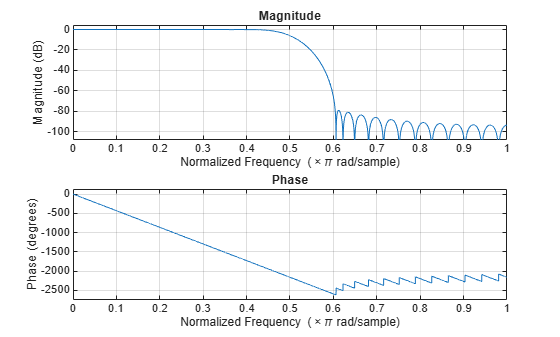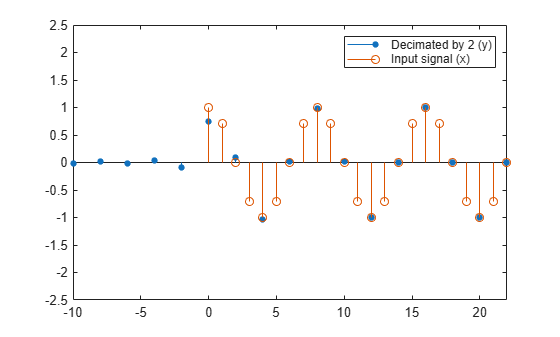Documentation

# dsp.FIRDecimator

Polyphase FIR decimator

## Description

The `dsp.FIRDecimator` System object™ resamples vector or matrix inputs along the first dimension. The object resamples at a rate M times slower than the input sampling rate, where M is the integer-valued downsampling factor. The decimation combines an FIR anti-aliasing filter with downsampling. The FIR decimator object uses a polyphase implementation of the FIR filter.

To resample vector or matrix inputs along the first dimension:

1. Create the `dsp.FIRDecimator` object and set its properties.

2. Call the object with arguments, as if it were a function.

To learn more about how System objects work, see What Are System Objects? (MATLAB).

## Creation

### Syntax

``firdecim = dsp.FIRDecimator``
``firdecim = dsp.FIRDecimator(decimFactor,num)``
``firdecim = dsp.FIRDecimator(___,Name,Value)``

### Description

example

````firdecim = dsp.FIRDecimator` returns an FIR decimator, `firdecim`, which applies an FIR filter with a cutoff frequency of `0.4*pi` radians/sample to the input and downsamples the filter output by factor of 2.```
````firdecim = dsp.FIRDecimator(decimFactor,num)` returns an FIR decimator with the integer-valued `DecimationFactor` property set to `decimFactor` and the `Numerator` property set to `num`.```
````firdecim = dsp.FIRDecimator(___,Name,Value)` returns an FIR decimator object with each specified property set to the specified value. Enclose each property name in quotes. You can use this syntax with any previous input argument combinations.```

## Properties

expand all

Unless otherwise indicated, properties are nontunable, which means you cannot change their values after calling the object. Objects lock when you call them, and the `release` function unlocks them.

If a property is tunable, you can change its value at any time.

For more information on changing property values, see System Design in MATLAB Using System Objects (MATLAB).

Decimation factor, specified as a positive integer. The FIR decimator reduces the sampling rate of the input by this factor. The number of input rows must be a multiple of the decimation factor.

Data Types: `single` | `double` | `int8` | `int16` | `int32` | `int64` | `uint8` | `uint16` | `uint32` | `uint64`

FIR filter coefficient source, specified as either:

• `'Property'` –– The numerator coefficients are specified through the `Numerator` property.

• `'Input port'` –– The numerator coefficients are specified as an input to the object algorithm.

Specify the numerator coefficients of the FIR filter in powers of z–1. The following equation defines the system function for a filter of length L:

`$H\left(z\right)=\sum _{l=0}^{L-1}{b}_{l}{z}^{-l}$`

To prevent aliasing as a result of downsampling, the filter transfer function should have a normalized cutoff frequency no greater than 1/`DecimationFactor`. You can specify the filter coefficients as a vector in the supported data types.

#### Dependencies

This property applies when `NumeratorSource` is set to `'Property'`.

Data Types: `single` | `double` | `int8` | `int16` | `int32` | `int64` | `uint8` | `uint16` | `uint32` | `uint64`
Complex Number Support: Yes

Specify the implementation of the FIR filter as either ```Direct form``` or `Direct form transposed`.

### Fixed-Point Properties

Flag to use full-precision rules for fixed-point arithmetic, specified as one of the following:

• `true` –– The object computes all internal arithmetic and output data types using the full-precision rules. These rules provide the most accurate fixed-point numerics. In this mode, other fixed-point properties do not apply. No quantization occurs within the object. Bits are added, as needed, to ensure that no roundoff or overflow occurs.

• `false` –– Fixed-point data types are controlled through individual fixed-point property settings.

For more information, see Full Precision for Fixed-Point System Objects and Set System Object Fixed-Point Properties.

Rounding method for fixed-point operations. For more details, see rounding mode.

#### Dependencies

This property is not visible and has no effect on the numerical results when the following conditions are met:

• `FullPrecisionOverride` set to `true`.

• `FullPrecisionOverride` set to `false`, `ProductDataType` set to `'Full precision'`, `AccumulatorDataType` set to ```'Full precision'```, and `OutputDataType` set to `'Same as accumulator'`.

Under these conditions, the object operates in full precision mode.

Overflow action for fixed-point operations, specified as one of the following:

• `'Wrap'` –– The object wraps the result of its fixed-point operations.

• `'Saturate'` –– The object saturates the result of its fixed-point operations.

For more details on overflow actions, see overflow mode for fixed-point operations.

#### Dependencies

This property is not visible and has no effect on the numerical results when the following conditions are met:

• `FullPrecisionOverride` set to `true`.

• `FullPrecisionOverride` set to `false`, `OutputDataType` set to `'Same as accumulator'`, `ProductDataType` set to ```'Full precision'```, and `AccumulatorDataType` set to `'Full precision'`

Under these conditions, the object operates in full precision mode.

Data type of the FIR filter coefficients, specified as:

• `Same word length as input` –– The word length of the coefficients is the same as that of the input. The fraction length is computed to give the best possible precision.

• `Custom` –– The coefficients data type is specified as a custom numeric type through the `CustomCoefficientsDataType` property.

Word and fraction lengths of the coefficients data type, specified as an autosigned `numerictype` with a word length of 16 and a fraction length of 15.

#### Dependencies

This property applies when you set the `CoefficientsDataType` property to `Custom`.

Data type of the product output in this object, specified as one of the following:

• `'Full precision'` –– The product output data type has full precision.

• `'Same as input'` –– The object specifies the product output data type to be the same as that of the input data type.

• `'Custom'` –– The product output data type is specified as a custom numeric type through the `CustomProductDataType` property.

For more information on the product output data type, see Multiplication Data Types.

#### Dependencies

This property applies when you set `FullPrecisionOverride` to `false`.

Word and fraction lengths of the product data type, specified as an autosigned numeric type with a word length of 32 and a fraction length of 30.

#### Dependencies

This property applies only when you set `FullPrecisionOverride` to `false` and `ProductDataType` to `'Custom'`.

Data type of an accumulation operation in this object, specified as one of the following:

• `'Full precision'` –– The accumulation operation has full precision.

• `'Same as product'` –– The object specifies the accumulator data type to be the same as that of the product output data type.

• `'Same as input'` –– The object specifies the accumulator data type to be the same as that of the input data type.

• `'Custom'` –– The accumulator data type is specified as a custom numeric type through the `CustomAccumulatorDataType` property.

#### Dependencies

This property applies when you set `FullPrecisionOverride` to `false`.

Word and fraction lengths of the accumulator data type, specified as an autosigned numeric type with a word length of 32 and a fraction length of 30.

#### Dependencies

This property applies only when you set `FullPrecisionOverride` to `false` and `AccumulatorDataType` to `'Custom'`.

Data type of the object output, specified as one of the following:

• `'Same as accumulator'` –– The output data type is the same as that of the accumulator output data type.

• `'Same as input'` –– The output data type is the same as that of the input data type.

• `'Same as product'` –– The output data type is the same as that of the product output data type.

• `'Custom'` –– The output data type is specified as a custom numeric type through the `CustomOutputDataType` property.

#### Dependencies

This property applies when you set `FullPrecisionOverride` to `false`.

Word and fraction lengths of the output data type, specified as an autosigned numeric type with a word length of 16 and a fraction length of 15.

#### Dependencies

This property applies only when you set `FullPrecisionOverride` to `false` and `OutputDataType` to `'Custom'`.

## Usage

### Syntax

``y = firdecim(x)``
``y = firdecim(x,num)``

### Description

example

````y = firdecim(x)` outputs the filtered and downsampled values, `y`, of the input signal, `x`.```
````y = firdecim(x,num)` uses the FIR filter, `num`, to decimate the input signal. This configuration is valid only when the `'NumeratorSource'` property is set to `'Input port'`.```

### Input Arguments

expand all

Data input, specified as a column vector or a matrix of size Ki-by-N. The number of input rows, Ki, must be a multiple of the `DecimationFactor` property. The input columns represent the N independent channels.

This object supports variable-size input and does not support complex unsigned fixed-point inputs.

Data Types: `single` | `double` | `int8` | `int16` | `int32` | `int64` | `uint8` | `uint16` | `uint32` | `uint64` | `fi`
Complex Number Support: Yes

FIR filter coefficients, specified as a row vector.

#### Dependencies

This input is accepted only when the `'NumeratorSource'` property is set to `'Input port'`.

Data Types: `double`
Complex Number Support: Yes

### Output Arguments

expand all

FIR decimated output, returned as a column vector or a matrix of size Ki/M-by-N, where M is the decimation factor.

Data Types: `single` | `double` | `int8` | `int16` | `int32` | `int64` | `uint8` | `uint16` | `uint32` | `uint64` | `fi`
Complex Number Support: Yes

## Object Functions

To use an object function, specify the System object as the first input argument. For example, to release system resources of a System object named `obj`, use this syntax:

`release(obj)`

expand all

 `freqz` Frequency response of filter `fvtool` Visualize frequency response of DSP filters `info` Information about filter System object `cost` Estimate cost for implementing filter System objects `polyphase` Polyphase decomposition of multirate filter `generatehdl` Generate HDL code for quantized DSP filter (requires Filter Design HDL Coder) `impz` Impulse response of discrete-time filter System object `coeffs` Filter coefficients
 `step` Run System object algorithm `release` Release resources and allow changes to System object property values and input characteristics `reset` Reset internal states of System object

## Examples

expand all

Note: This example runs only in R2016b or later. If you are using an earlier release, replace each call to the function with the equivalent `step` syntax. For example, myObject(x) becomes step(myObject,x).

This example shows how to decimate a sum of sine waves with angular frequencies of pi/4 and 2pi/3 radians/sample by a factor of two. To prevent aliasing, the FIR decimator filters out the 2pi/3 radians/sample component before downsampling.

```x = cos(pi/4*(0:95)')+sin(2*pi/3*(0:95)'); firdecim = dsp.FIRDecimator; y = firdecim(x);```

View group delay of default FIR filter

`fvtool(fir1(35,0.4),1,'analysis','grpdelay');`Group delay of the default linear-phase FIR filter is 17.5 samples. Downsampling by a factor of two expect an approx. 8.75 sample delay in the output y with the initial filter states of zero

```subplot(211); stem(x(1:length(x)/2),'b','markerfacecolor',[0 0 1]); title('Input Signal'); subplot(212); stem(y,'b','markerfacecolor',[0 0 1]); title('Output--Lowpass filtered and downsampled by 2');```Note: If you are using R2016a or an earlier release, replace each call to the object with the equivalent `step `syntax. For example, `obj(x) `becomes `step(obj,x)`.

Note: The `dsp.AudioFileReader` and `audioDeviceWriter` System objects are not supported in MATLAB Online.

This example shows how to reduce the sampling rate of an audio signal by 1/2 and plays it.

```afr = dsp.AudioFileReader('OutputDataType',... 'single'); adw = audioDeviceWriter(22050/2); firdecim = dsp.FIRDecimator; while ~isDone(afr) frame = afr(); y = firdecim(frame); adw(y); end release(afr); pause(0.5); release(adw);```

expand all

## Algorithms

This object implements the algorithm, inputs, and outputs described on the FIR Decimation block reference page. The object properties correspond to the block parameters, except:

• Framing – The FIR decimator object only supports `Maintain input frame rate`

• Output buffer initial conditions – The FIR decimator object does not support this parameter.

• Rate options – The FIR decimator object does not support this parameter.

• Input processing The FIR decimator object does not support this parameter.

Watch now